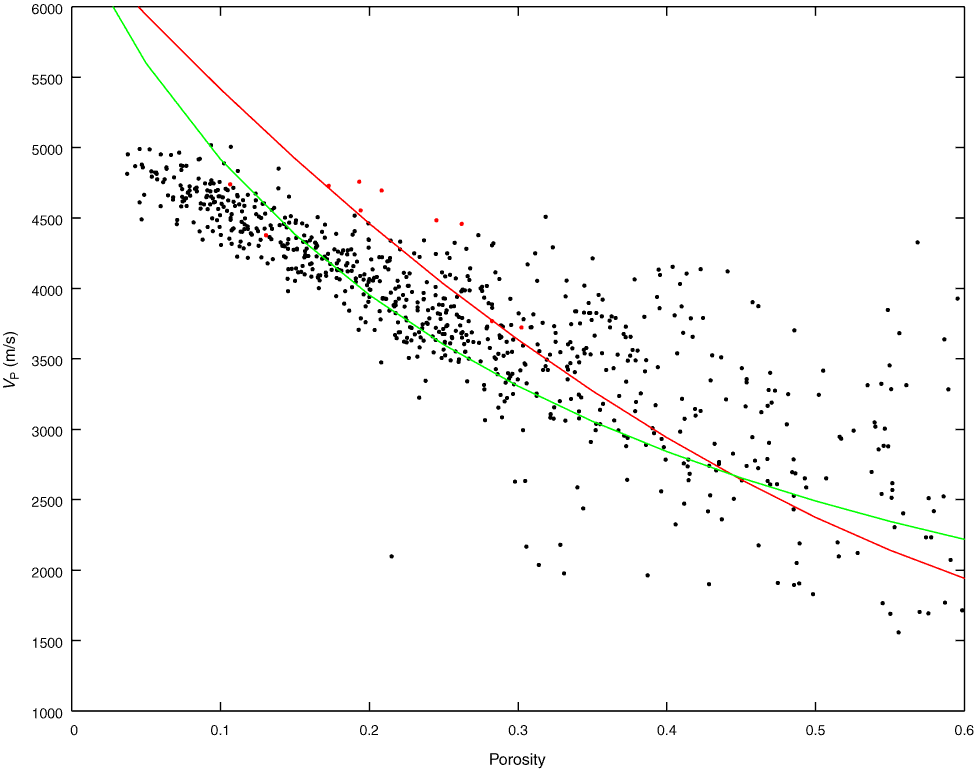Figure F68. Cross plot of porosity with velocity for Hole M0017A. Solid lines refer to the Wyllie time average equation (red) and Raymer modified time average equation (green) for a matrix velocity of calcite (6530 m/s). Discrete measurements are superimposed (red circles).